Miscellaneous

Chapter 4 Class 12 Determinants
Serial order wise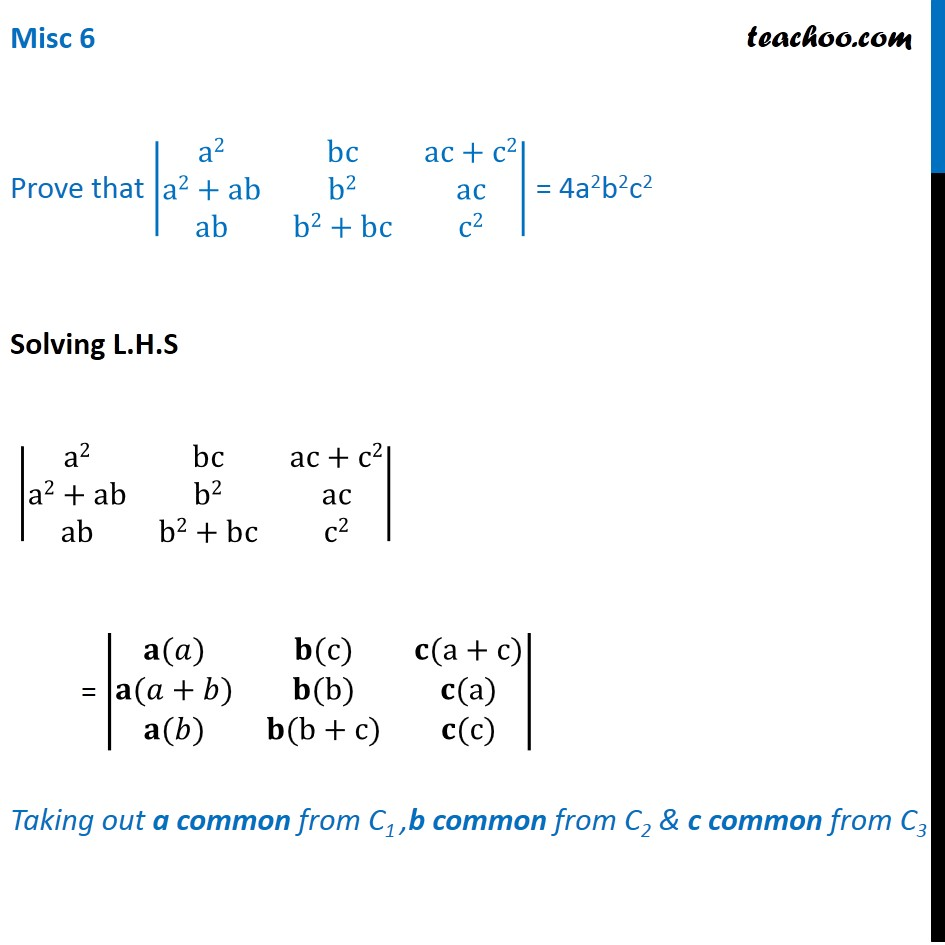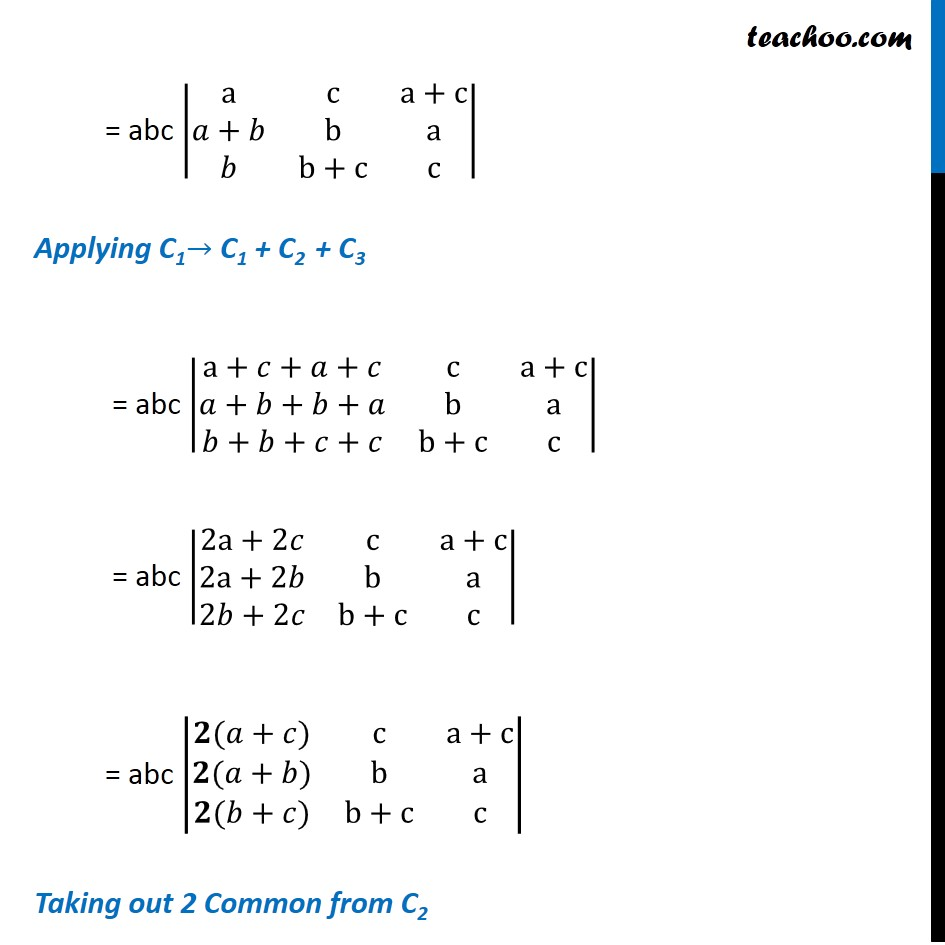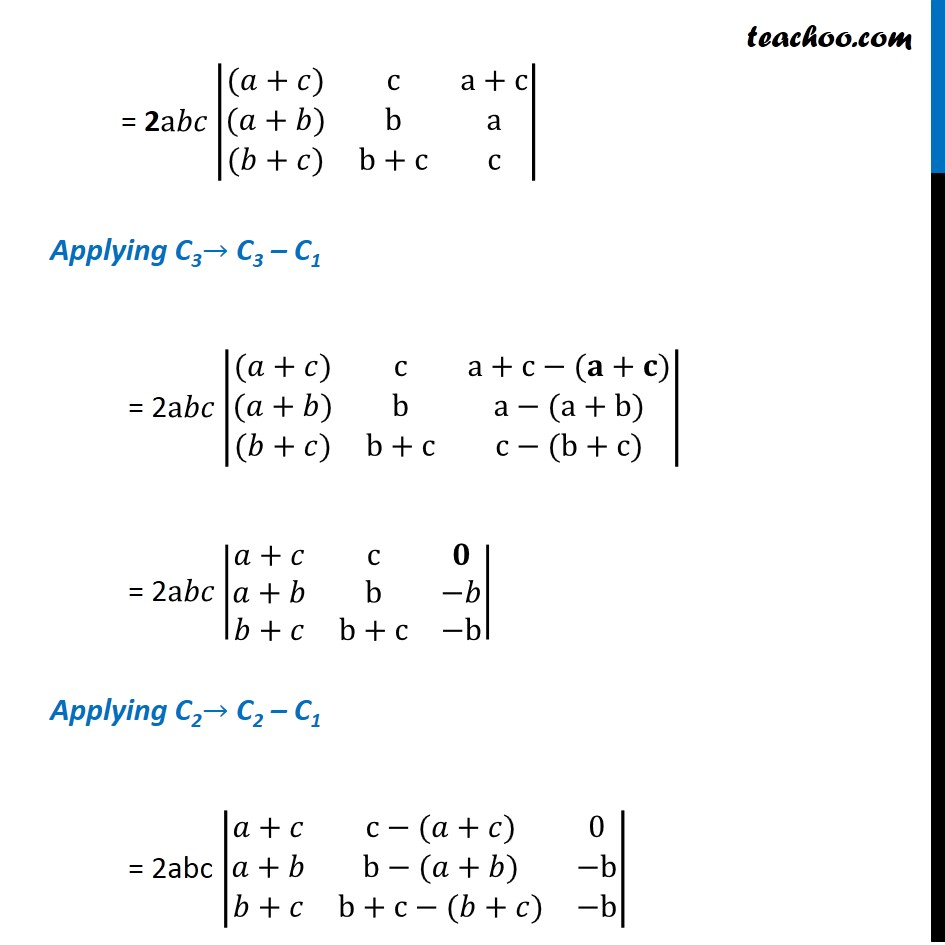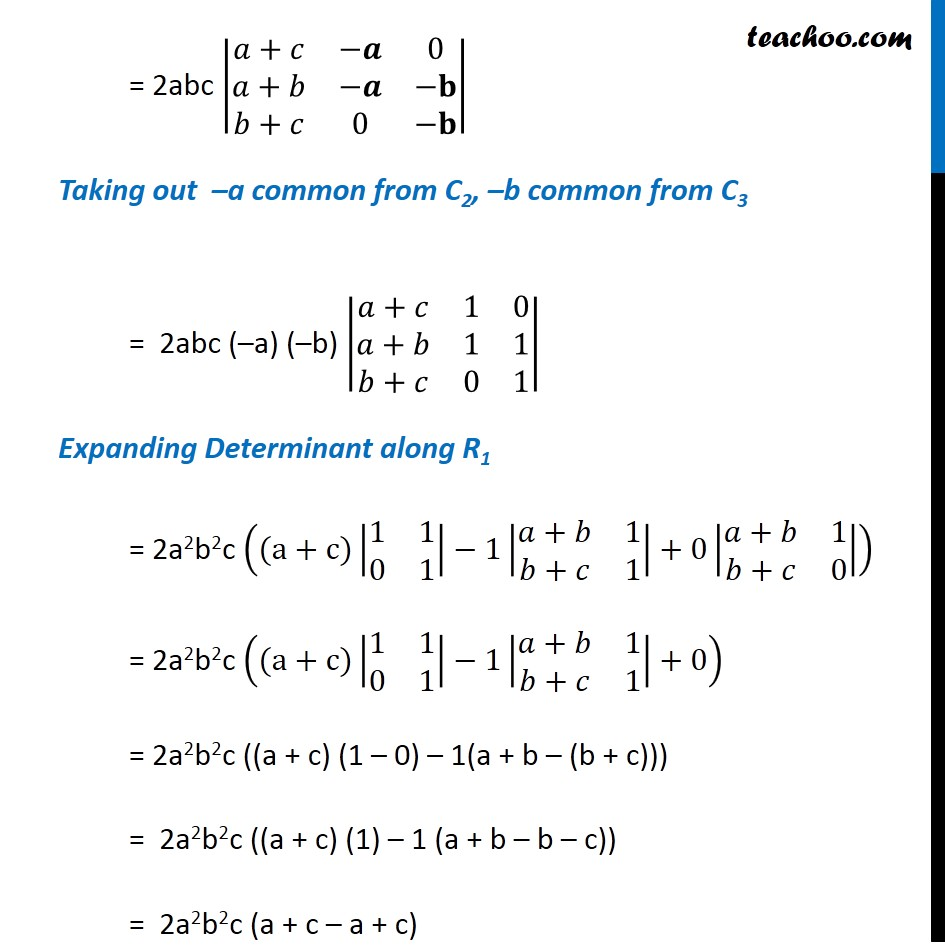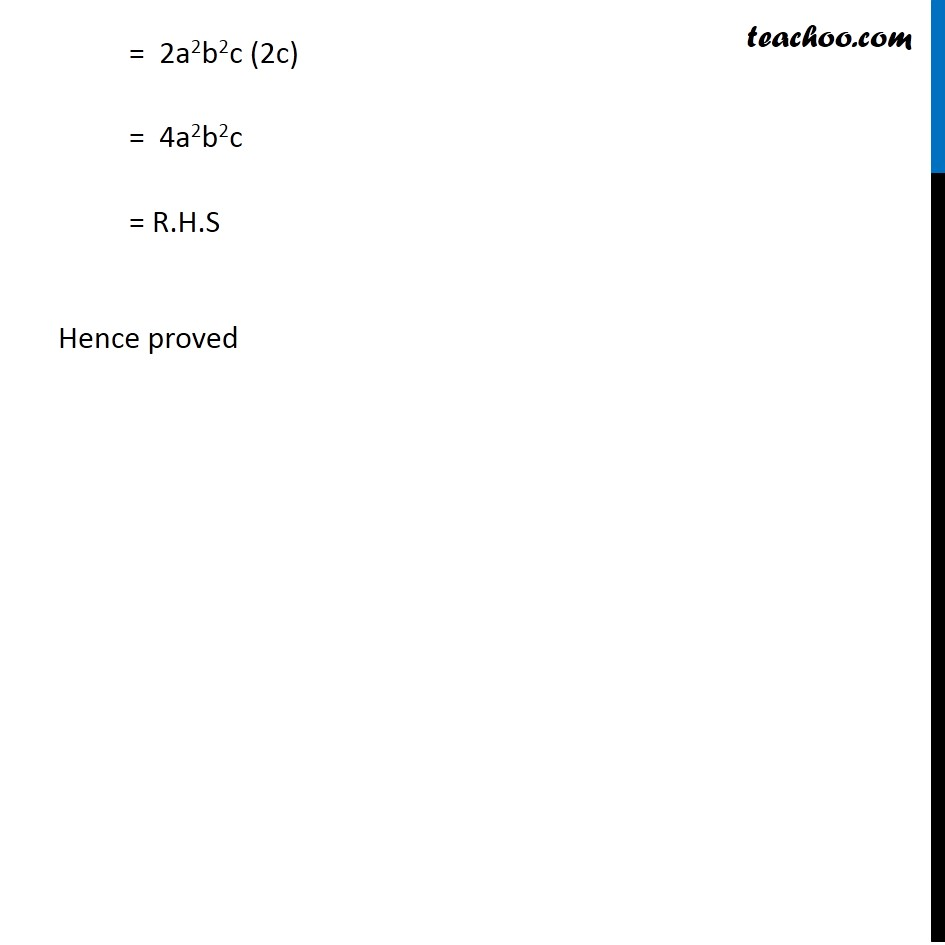Learn in your speed, with individual attention - Teachoo Maths 1-on-1 Class

### Transcript

Question 4 Prove that |■8(a2&bc&ac+c2@a2+ab&b2&ac@ab&b2+bc&c2)| = 4a2b2c2 Solving L.H.S |■8(a2&bc&ac+c2@a2+ab&b2&ac@ab&b2+bc&c2)| = |■8(𝐚(𝑎)&𝐛(c)&𝐜(a+c)@𝐚(𝑎+𝑏)&𝐛(b)&𝐜(a)@𝐚(𝑏)&𝐛(b+c)&𝐜(c))| Taking out a common from C1 ,b common from C2 & c common from C3 = abc |■8(a&c&a+c@𝑎+𝑏&b&a@𝑏&b+c&c)| Applying C1→ C1 + C2 + C3 = abc |■8(a+𝑐+𝑎+𝑐&c&a+c@𝑎+𝑏+𝑏+𝑎&b&a@𝑏+𝑏+𝑐+𝑐&b+c&c)| = abc |■8(2a+2𝑐&c&a+c@2a+2𝑏&b&a@2𝑏+2𝑐&b+c&c)| = abc |■8(𝟐(𝑎+𝑐)&c&a+c@𝟐(𝑎+𝑏)&b&a@𝟐(𝑏+𝑐)&b+c&c)| Taking out 2 Common from C2 = 2a𝑏𝑐|■8((𝑎+𝑐)&c&a+c@(𝑎+𝑏)&b&a@(𝑏+𝑐)&b+c&c)| Applying C3→ C3 – C1 = 2a𝑏𝑐|■8((𝑎+𝑐)&c&a+c−(𝐚+𝐜)@(𝑎+𝑏)&b&a−(a+b)@(𝑏+𝑐)&b+c&c−(b+c))| = 2a𝑏𝑐|■8(𝑎+𝑐&c&𝟎@𝑎+𝑏&b&−𝑏@𝑏+𝑐&b+c&−b)| Applying C2→ C2 – C1 = 2abc |■8(𝑎+𝑐&c−(𝑎+𝑐)&0@𝑎+𝑏&b−(𝑎+𝑏)&−b@𝑏+𝑐&b+c−(𝑏+𝑐)&−b)| = 2abc |■8(𝑎+𝑐&−𝒂&0@𝑎+𝑏&−𝒂&−𝐛@𝑏+𝑐&0&−𝐛)| Taking out –a common from C2, –b common from C3 = 2abc (–a) (–b) |■8(𝑎+𝑐&1&0@𝑎+𝑏&1&1@𝑏+𝑐&0&1)| Expanding Determinant along R1 = 2a2b2c ((a+c)|■8(1&1@0&1)|−1|■8(𝑎+𝑏&1@𝑏+𝑐&1)|+0|■8(𝑎+𝑏&1@𝑏+𝑐&0)|) = 2a2b2c ((a+c)|■8(1&1@0&1)|−1|■8(𝑎+𝑏&1@𝑏+𝑐&1)|+0) = 2a2b2c ((a + c) (1 – 0) – 1(a + b – (b + c))) = 2a2b2c ((a + c) (1) – 1 (a + b – b – c)) = 2a2b2c (a + c – a + c) = 2a2b2c (2c) = 4a2b2c = R.H.S Hence proved